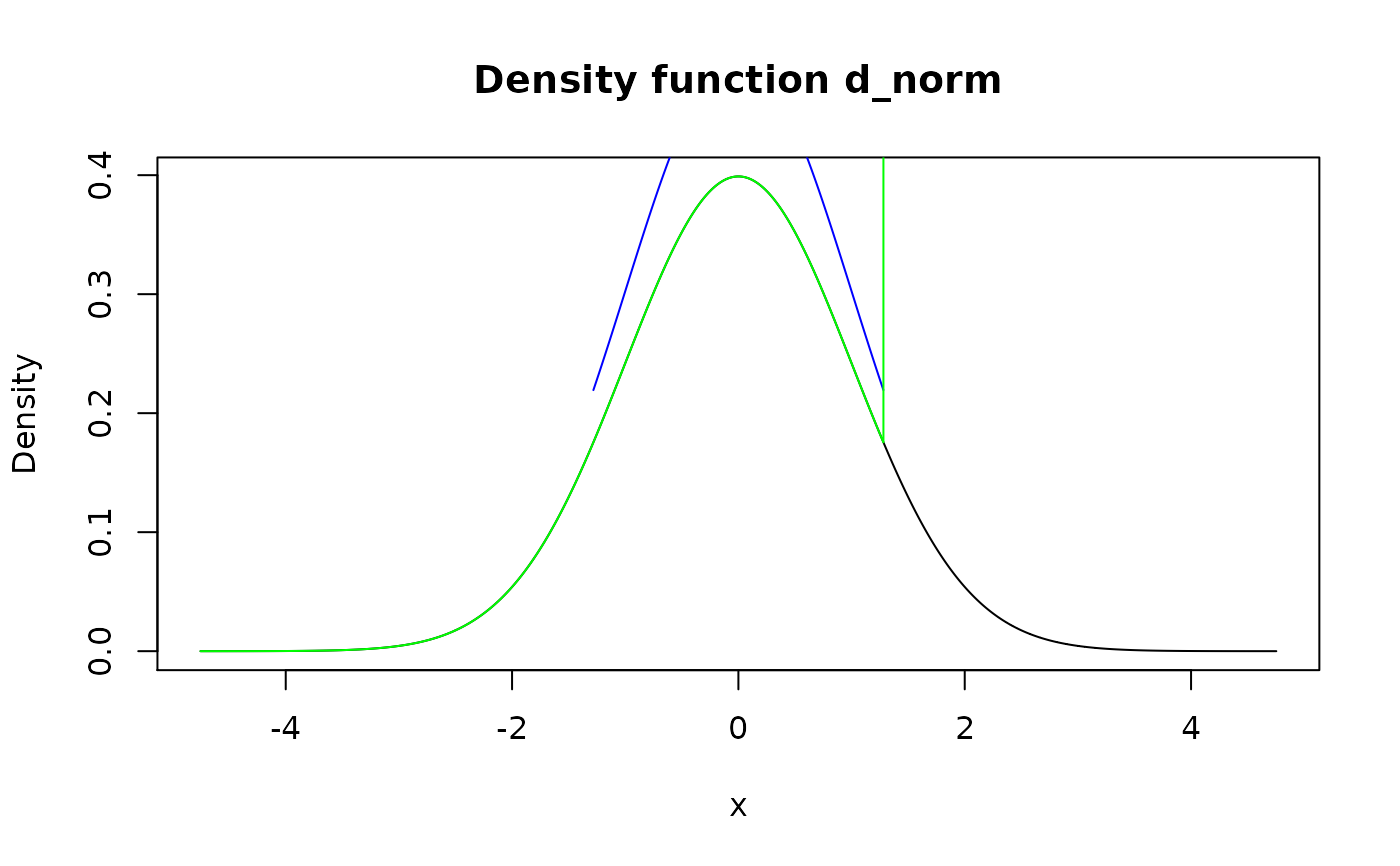Modify tail(s) of distribution defined by certain cutoff level using method of choice. This function is useful for doing robust analysis in presence of possible outliers.

form_tails(f, level, method = "trim", direction = "both")

Arguments

f A pdqr-function. Cutoff level. For direction "both" should be between 0 and 0.5; for "left" and "right" - between 0 and 1. Modification method. One of "trim" or "winsor". Information about which tail(s) to modify. One of "both", "left", "right".

Value

A pdqr-function with transformed tail(s).

Details

Edges for left and right tails are computed as level and 1 - level quantiles respectively. The left tail is interval to the left of left edge, and right tail - to the right of right edge.

Method "trim" removes tail(s) while normalizing "center part". Method "winsor" "squashes" tails inside center of distribution in dirac-like fashion, i.e. probability of tail(s) is moved inside and becomes concentrated in 1e-8 neighborhood of nearest edge.

Direction "both" affect both tails. Directions "left" and "right" affect only left and right tail respectively.

form_resupport() for changing support to some known interval.

summ_center() and summ_spread() for computing summaries of distributions.

Other form functions: form_estimate(), form_mix(), form_regrid(), form_resupport(), form_retype(), form_smooth(), form_trans()

Examples

# Type "discrete" my_dis <- new_d(data.frame(x = 1:4, prob = (1:4) / 10), type = "discrete") meta_x_tbl(form_tails(my_dis, level = 0.1))
#> x prob cumprob #> 1 2 0.250 0.250 #> 2 3 0.375 0.625 #> 3 4 0.375 1.000
meta_x_tbl( form_tails(my_dis, level = 0.35, method = "winsor", direction = "left") )
#> x prob cumprob #> 1 3 0.6 0.6 #> 2 4 0.4 1.0
# Type "continuous" d_norm <- as_d(dnorm) plot(d_norm)
lines(form_tails(d_norm, level = 0.1), col = "blue")
lines( form_tails(d_norm, level = 0.1, method = "winsor", direction = "right"), col = "green" )# Use form_resupport() and as_q() to remove different levels from both # directions. Here 0.1 level tail from left is removed, and 0.05 level from # right new_supp <- as_q(d_norm)(c(0.1, 1 - 0.05)) form_resupport(d_norm, support = new_supp)
#> Density function of continuous type #> Support: ~[-1.28155, 1.64485] (9236 intervals)
# Examples of robust mean set.seed(101) x <- rcauchy(1000) d_x <- new_d(x, "continuous") summ_mean(d_x)
#>  0.6504152
## Trimmed mean summ_mean(form_tails(d_x, level = 0.1, method = "trim"))
#>  0.1094364
## Winsorized mean summ_mean(form_tails(d_x, level = 0.1, method = "winsor"))
#>  0.1563657